Test: Functions Of One,Two Or Three Real Variables - 6

# Test: Functions Of One,Two Or Three Real Variables - 6

Test Description

## 20 Questions MCQ Test Topic-wise Tests & Solved Examples for IIT JAM Mathematics | Test: Functions Of One,Two Or Three Real Variables - 6

Test: Functions Of One,Two Or Three Real Variables - 6 for Mathematics 2023 is part of Topic-wise Tests & Solved Examples for IIT JAM Mathematics preparation. The Test: Functions Of One,Two Or Three Real Variables - 6 questions and answers have been prepared according to the Mathematics exam syllabus.The Test: Functions Of One,Two Or Three Real Variables - 6 MCQs are made for Mathematics 2023 Exam. Find important definitions, questions, notes, meanings, examples, exercises, MCQs and online tests for Test: Functions Of One,Two Or Three Real Variables - 6 below.
Solutions of Test: Functions Of One,Two Or Three Real Variables - 6 questions in English are available as part of our Topic-wise Tests & Solved Examples for IIT JAM Mathematics for Mathematics & Test: Functions Of One,Two Or Three Real Variables - 6 solutions in Hindi for Topic-wise Tests & Solved Examples for IIT JAM Mathematics course. Download more important topics, notes, lectures and mock test series for Mathematics Exam by signing up for free. Attempt Test: Functions Of One,Two Or Three Real Variables - 6 | 20 questions in 60 minutes | Mock test for Mathematics preparation | Free important questions MCQ to study Topic-wise Tests & Solved Examples for IIT JAM Mathematics for Mathematics Exam | Download free PDF with solutions
 1 Crore+ students have signed up on EduRev. Have you?
Test: Functions Of One,Two Or Three Real Variables - 6 - Question 1

### A saltus at point of continuity is equal to

Test: Functions Of One,Two Or Three Real Variables - 6 - Question 2

### Which of the following functions f: Z X Z → Z is not onto?

Detailed Solution for Test: Functions Of One,Two Or Three Real Variables - 6 - Question 2

The function is not onto as f(a) ≠ b.

Test: Functions Of One,Two Or Three Real Variables - 6 - Question 3

### Assertion (A) : If is continuous on [a ,b] then there exists a real number u such that f(x) ≤ u,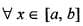Reason (R) : If is continuous in [a,b] then it attains its bounds in [a, b].

Test: Functions Of One,Two Or Three Real Variables - 6 - Question 4

Let f be defined for all real x such that |f(x) - f(y)| < (x - y)2 for all real x and y, then

Test: Functions Of One,Two Or Three Real Variables - 6 - Question 5

What is the domain and range of f?.

Where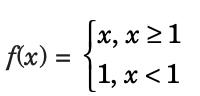Detailed Solution for Test: Functions Of One,Two Or Three Real Variables - 6 - Question 5

Here, function is defined in stages, for x ≥ 1: the formula y = x is defined for all values of x greater than or equal to unity. The domain for this part is [1, ∞].
For x < 1 is defined for all values less than unity. Then the domain of this part is (– ∞ ,1).
Hence, domain of given function is (– ∞ ,1) ∪ [1, ∞) = (– ∞, ∞).

Test: Functions Of One,Two Or Three Real Variables - 6 - Question 6

Let f(x, y) = xy then,

Test: Functions Of One,Two Or Three Real Variables - 6 - Question 7

For what value of k, the function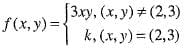is continuous?

Test: Functions Of One,Two Or Three Real Variables - 6 - Question 8

For function f(x,y) to have minimum value at (a,b) value is?

Detailed Solution for Test: Functions Of One,Two Or Three Real Variables - 6 - Question 8

For the function f(x,y) to have minimum value at (a,b)
rt – s2>0 and r>0
where, r = 2f∂x2, t=2f∂y2, s=2f∂x∂y, at (x,y) => (a,b)

Test: Functions Of One,Two Or Three Real Variables - 6 - Question 9

Let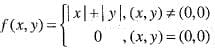then,

Test: Functions Of One,Two Or Three Real Variables - 6 - Question 10

If f (x) =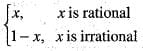then which of the following is incorrect?

Test: Functions Of One,Two Or Three Real Variables - 6 - Question 11

An example of a function on the real line R i.e., continuous but not uniformly continuous is

Test: Functions Of One,Two Or Three Real Variables - 6 - Question 12

Let f: [0, 10] → [0, 10] be a continuous mapping, then

Test: Functions Of One,Two Or Three Real Variables - 6 - Question 13

For the function f (x) defined as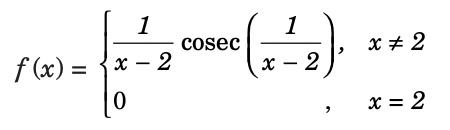Detailed Solution for Test: Functions Of One,Two Or Three Real Variables - 6 - Question 13

RHL: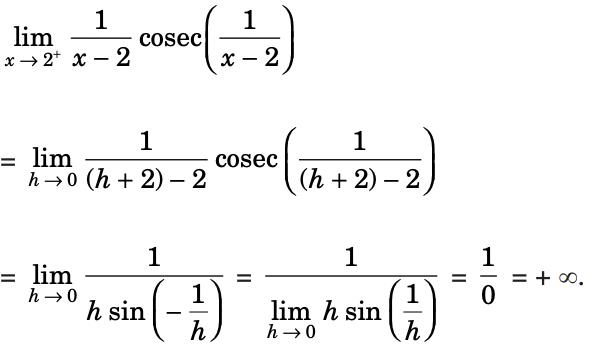LHL: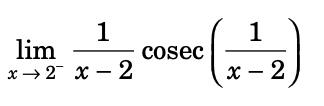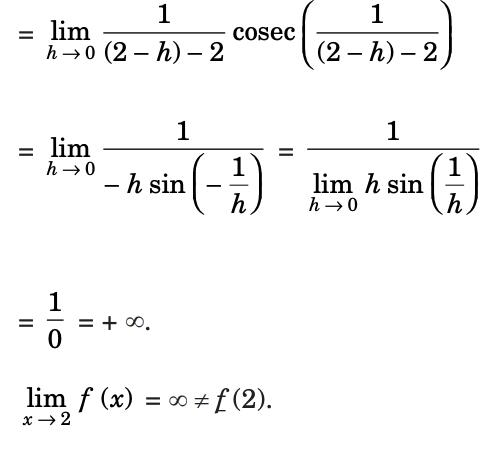So, the function is not continuous at the point x = 2 and having an infinite discontinuity of the second kind.

Test: Functions Of One,Two Or Three Real Variables - 6 - Question 14

Under which one of the following conditions does the function f(x) = [(x2)m sin (x-2)n], x ≠ 0, n > 0 and f(0) = 0, have a derivative at x = 0?

Test: Functions Of One,Two Or Three Real Variables - 6 - Question 15

The value of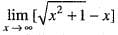is

Test: Functions Of One,Two Or Three Real Variables - 6 - Question 16

Suppose f: [a, b] → R is continuous on [a, b] and f is differentiable on (a, b). If f(a) = f(b), there is c ∈ (a,b)-.f'(c) = 0

Test: Functions Of One,Two Or Three Real Variables - 6 - Question 17

Let y be continuously differentiable function which satisfies the differential equation
y" + y' - y = 0,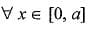where a is a positive real number, if y(0) = y(a) - 0, then on [0, a].

Test: Functions Of One,Two Or Three Real Variables - 6 - Question 18

If f(x) =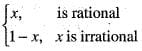then which of the following is incorrect?

Test: Functions Of One,Two Or Three Real Variables - 6 - Question 19

If f is decreasing function on E ⊂ R, then for x, y ∈ E, We have

Test: Functions Of One,Two Or Three Real Variables - 6 - Question 20

Which one is uniformly continuous in [0, ∞]?

## Topic-wise Tests & Solved Examples for IIT JAM Mathematics

27 docs|150 tests
 Use Code STAYHOME200 and get INR 200 additional OFF Use Coupon Code
Information about Test: Functions Of One,Two Or Three Real Variables - 6 Page
In this test you can find the Exam questions for Test: Functions Of One,Two Or Three Real Variables - 6 solved & explained in the simplest way possible. Besides giving Questions and answers for Test: Functions Of One,Two Or Three Real Variables - 6, EduRev gives you an ample number of Online tests for practice

## Topic-wise Tests & Solved Examples for IIT JAM Mathematics

27 docs|150 tests